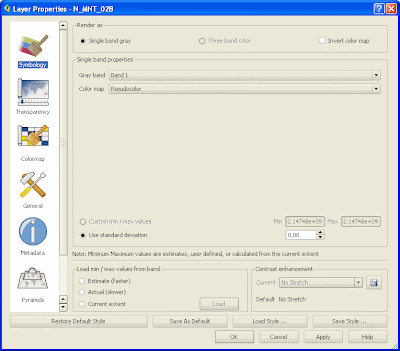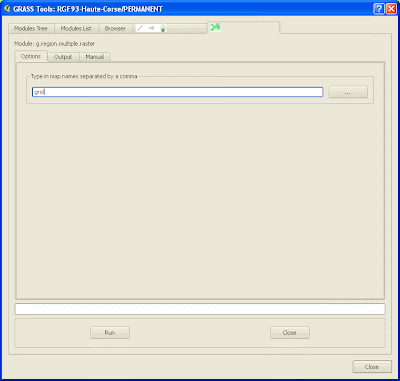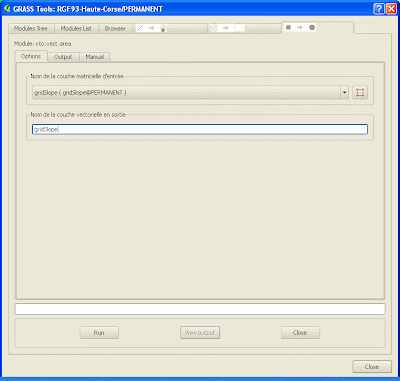# [Rasters in QGIS] Generate a Vector Grid with Average Slope Values

Operations:

• Slope: DEM Layer.tif=[QGIS/Raster Based Terrain Analysis]=>slope.tif=>[r.in.gdal]=>slope
• Grid: [QGIS/Vector/Research Tools/Vector Grid]=>grid.shp=[v.in.ogr]=>grid=>[v.to.rast]=>grid
• Crossing Grid and Slope: grid*slope=[r.average]=>gridSlope=[r.to.vect]=>gridSlope=[v.out.ogr]=>gridSlope.shpHere, we applied a pseudo-color tableSlope Raster creation
First, create a slope map using raster-based Terrain Raster Analysis PluginHere is the result with pseudo-color tableUse r.in.gdalVector Grid Creation
Create a grid covering your area of interest
Vector > Research Tools > Vector GridIt generates a grid shapefile with an ID Colum which is unique

Here, the grid covers the slope mapv.in.ogrRasterize your grid using ID Column
v.to.rast.attr
The ID attribute will become the cell’s categorySet the region on your rasterized vector gridGenerate an average slope grid using the two existing rasters
r.average
r.average – Finds the average of values in a cover map within areas assigned the same category value in a user-specified base map. “

Remember that each square area of the rasterized vector grid has a unique category as it corresponds to the previous ID attribute of the vector gridr.to.vect.areaHere, we labeled the generated vector using the cat column. Each number represents the average slope in each cell.Export it to shapeFile (optional)
v.out.ogrRemarks:
Slope could have been created using r.slope.aspect within GRASS instead of using QGIS plugin
You could apply the same procudure using features instead of a grid. For example, you could get the average slope touching and/or surrounding some urban areas in the context of a Terrain Movement study

Ce site utilise Akismet pour réduire les indésirables. En savoir plus sur comment les données de vos commentaires sont utilisées.